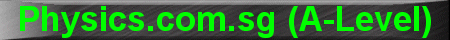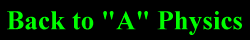(New tips are continually added to these pages.  Check back in a few months' time for more)

TOPIC 4:     Circular Motion and Gravitation

Tip 1:

When drawing the free-body-diagram for an object undergoing circular motion, do not add in the centripetal force.

The Centripetal Force is NOT a separate force on its own.  It is the resultant of all forces acting on the body.   It is the "F" in the equation F = ma.

In order for an object to undergo circular motion, it needs a centripetal force.  This centripetal force may be provided by SOME or ALL of the following: Tension, Weight, Friction, Reaction Force, Gravitational Pull, Electrostatic Attraction etc.

For example, a person sitting in a bus that is turning right around a circle will need a centripetal force to follow the bus round the circle.  If he cannot obtain the necessary centripetal force, he will not be able to go round the circle and may be flung out of the window.

In this case, the centripetal force comes from: the Reaction Force (R) from the left window on which he is leaning and the Friction (F) between his pants and the seat.  These two forces (R and F), both acting towards the right, together make up the centripetal force.  So the equation becomes: R + F = mv2/r.

Tip 2:

There are some popular questions in Gravitation regarding changes in speed of an object as it falls towards the earth.   This type of questions is best solved using the law of Conservation of Energy.

For example, if an object has a speed of v1 when it was at a distance of r1 from the centre of the Earth, and you are required to find its speed v2 when it has dropped to a distance of r2 from the centre of the Earth, apply the Conservation of Energy as follows:

Total Energy at Location 1 = Total Energy at Location 2

K.E.1 + P.E.1 = K.E.2 + P.E.2

˝ m v12 + (-GMm/r1) = ˝ m v22 + (-GMm/r2)

and solve for v2.

Notice that the mass of the object (m) is not required, since it cancels out on both sides of the equation.